﻿ Digital Circuits and Systems - Circuits i Sistemes Digitals (CSD) - EETAC - UPCBachelor's Degree in Telecommunications Systems and in Network Engineering## Simple timer circuits

RC, 555, and other timers

### 1. Timer circuits

The idea of a timer circuit is represented in Fig. 1. Slide.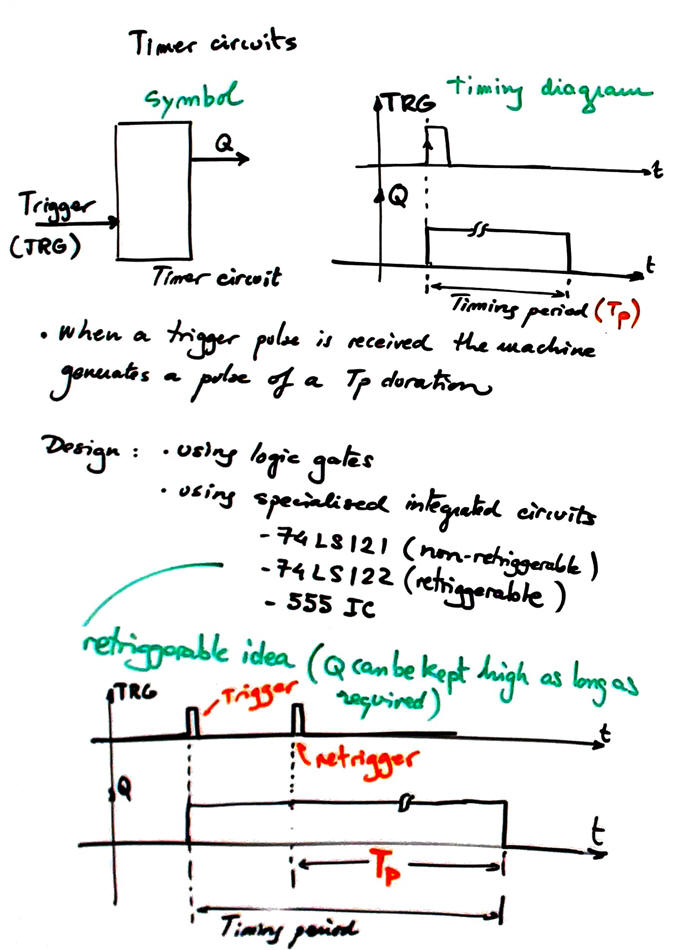Fig. 1. Symbol and operation of timer circuit. There is also for some circuits the extra retriggerable feature that allows enlarging timing period as much as desired.

### 2. Timer circuit based on logic gates

Fig. 2 shows the typical RC timer based on logic gates and on the idea of charging and discharging a capacitor. The analysis in Fig. 3 shows how to deduce its timing period (TP).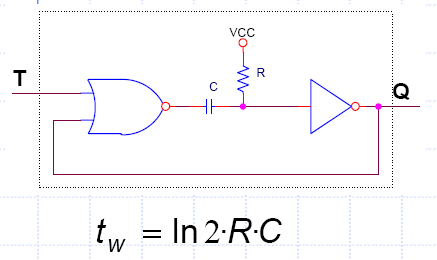Fig. 2. Typical RC timer. Time constant determines the circuit timing period along with technology values associated to low (NML) and high  (NMH) logic margins.

Analysis of this basic circuit is presented in Fig. 3.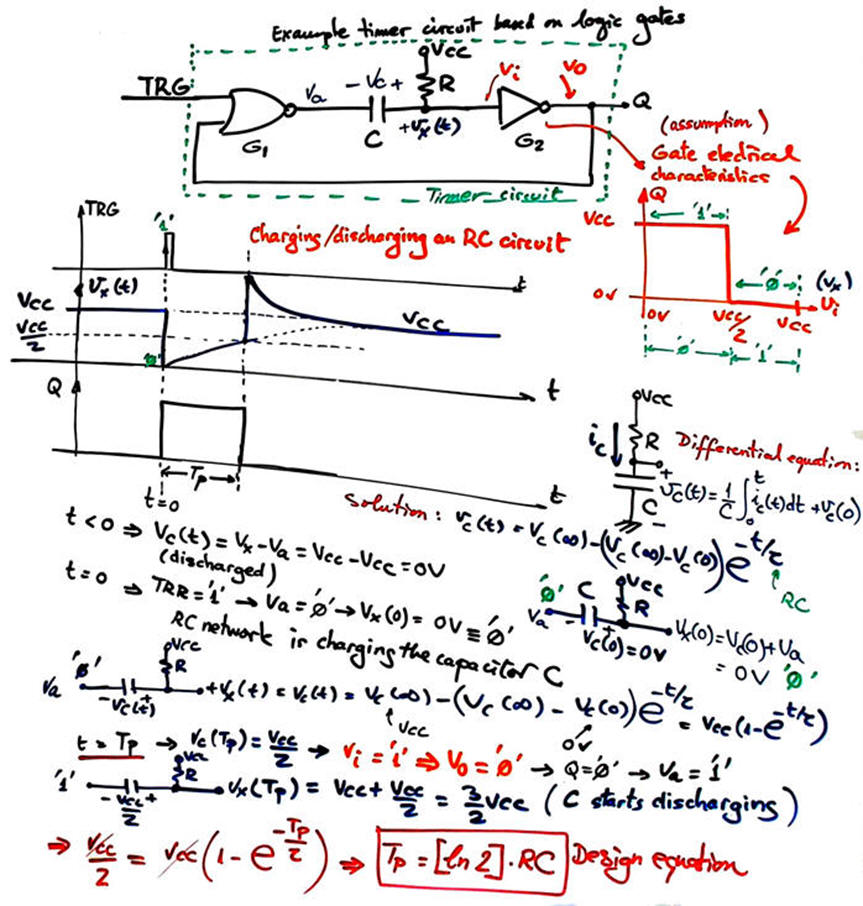Fig. 3. Analysis of a typical circuit based on logic gates. Let us assume that the transfer I/O characteristics of the logic gates are simplified as shown: no forbidden zone, but half of the input voltage margin interpreted as VIL, and the other half as VIH.

Typical classic timer integrated circuits are 74LS122 (retriggerable) and 74LS121 /74HCT221 (non retriggerable).

### 3. Timer circuit based on 555 chip

Fig. 4 shows the typical timer based on the 555 classic  circuit . This analysis shows how to deduce its timing period. This is the circuit 555_timer in Proteus.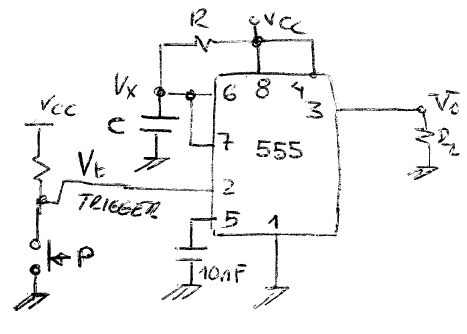Fig. 4. Typical timer based on 555 classic circuit.

Example application: Circuit simulator of the ultrasonic sensor HC-SR04: 555_distance_sensor.pdsprj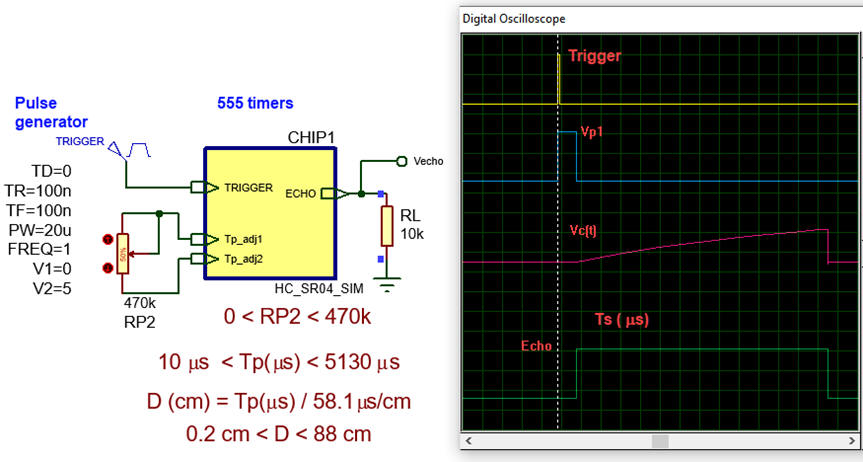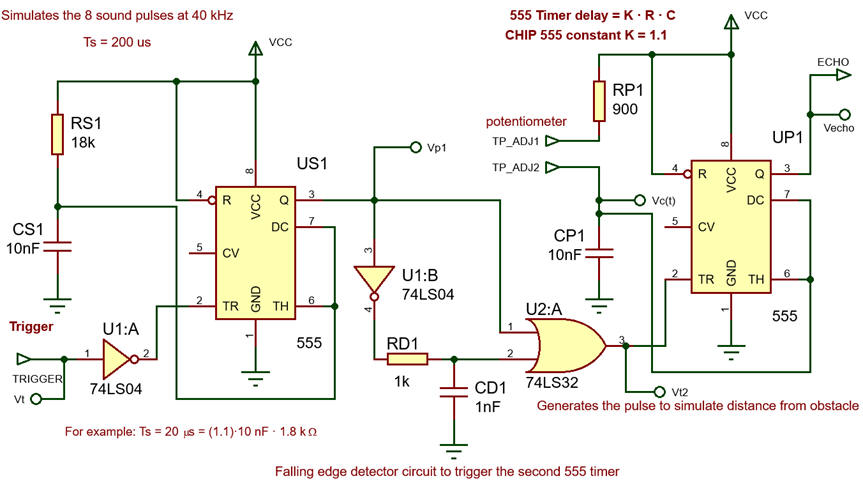Fig. 4. HC-SR04 ultrasonic Sensor Module simulator.